# Search Results (15)

View
Selected filters:
• CCSS.Math.Content.HSS-ID.A.4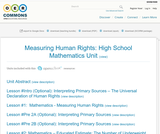Conditions of Use:
No Strings Attached
Rating

In this unit, students will read and interpret primary sources to address the question “How do we measure the attainment of human rights?” By exploring the Universal Declaration of Human Rights, the UN’s Guide to Indicators of Human Rights, and data about development indicators from multiple databases, students will unpack the complexities of using indicators to measure human rights.

Subject:
Mathematics
Material Type:
Assessment
Lesson Plan
Author:
Tamar Posner
Date Added:
01/28/2016
Remix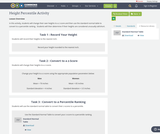Conditions of Use:
Remix and Share
Rating

In this activity, students will change their own heights to a z score and then use the standard normal table to convert to a percentile ranking.  Students will then determine if their heights are considered unusually tall/short.

Subject:
Educational Technology
Material Type:
Lesson Plan
Author:
Samantha Jensen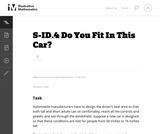Conditions of Use:
No Strings Attached
Rating

This task requires students to use the normal distribution as a model for a data distribution. Students must use given means and standard deviations to approximate population percentages. There are several ways (tables, graphing calculators, or statistical software) that students might calculate the required normal percentages. Depending on the method used, answers might vary somewhat from those shown in the solution.

Subject:
Mathematics
Material Type:
Activity/Lab
Provider:
Illustrative Mathematics
Provider Set:
Illustrative Mathematics
Author:
Illustrative Mathematics
Date Added:
01/02/2013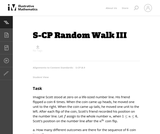Conditions of Use:
No Strings Attached
Rating

This task provides a context to calculate discrete probabilities and represent them on a bar graph. It could also be used to create a class activity where students gather, represent, and analyze data, running simulations of the random walk and recording and then displaying their results.

Subject:
Mathematics
Material Type:
Activity/Lab
Provider:
Illustrative Mathematics
Provider Set:
Illustrative Mathematics
Author:
Illustrative Mathematics
Date Added:
06/06/2012Conditions of Use:
Remix and Share
Rating

This problem could be used as an introductory lesson to introduce group comparisons and to engage students in a question they may find amusing and interesting. More generally, the idea of the lesson could be used as a template for a project where students develop a questionnaire, sample students at their school and report on their findings.

Subject:
Mathematics
Material Type:
Activity/Lab
Provider:
Illustrative Mathematics
Provider Set:
Illustrative Mathematics
Author:
Illustrative Mathematics
Date Added:
09/03/2012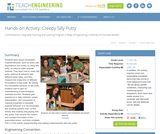Conditions of Use:
Read the Fine Print
Rating

Students learn about viscoelastic material behavior, such as strain rate dependence and creep, by using silly putty, an easy-to-make polymer material. They learn how to make silly putty, observe its behavior with different strain rates, and then measure the creep time of different formulations of silly putty. By seeing the viscoelastic behavior of silly putty, students start to gain an understanding of how biological materials function. Students gain experience in data collection, graph interpretation, and comparison of material properties to elucidate material behavior. It is recommended that students perform Part 1of the activity first (making and playing with silly putty), then receive the content and concept information in the associated lesson, and then complete Part 2 of the activity (experimenting and making measurements with silly putty).

Subject:
Architecture and Design
Engineering
Material Type:
Activity/Lab
Provider:
TeachEngineering
Provider Set:
TeachEngineering
Author:
Brandi N. Briggs
Denise W. Carlson
Marissa H. Forbes
Date Added:
09/18/2014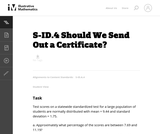Conditions of Use:
No Strings Attached
Rating

The purpose of this task is to have students complete normal distribution calculations and to use properties of normal distributions to draw conclusions. The task is designed to encourage students to communicate their findings in a narrative/report form in context Đ not just simply as a computed number.

Subject:
Mathematics
Material Type:
Activity/Lab
Provider:
Illustrative Mathematics
Provider Set:
Illustrative Mathematics
Author:
Illustrative Mathematics
Date Added:
12/26/2012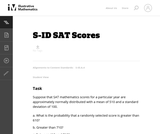Conditions of Use:
No Strings Attached
Rating

This task involving SAT scores requires students to calculate probability.

Subject:
Mathematics
Material Type:
Activity/Lab
Provider:
Illustrative Mathematics
Provider Set:
Illustrative Mathematics
Author:
Illustrative Mathematics
Date Added:
05/01/2012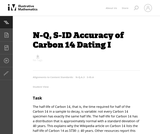Conditions of Use:
No Strings Attached
Rating

This task examines, from a mathematical and statistical point of view, how scientists measure the age of organic materials by measuring the ratio of Carbon 14 to Carbon 12. The focus here is on the statistical nature of such dating. This task addresses a very important issue about precision in reporting and understanding statements in a realistic scientific context.

Subject:
Mathematics
Material Type:
Activity/Lab
Provider:
Illustrative Mathematics
Provider Set:
Illustrative Mathematics
Author:
Illustrative Mathematics
Date Added:
09/13/2012Conditions of Use:
Read the Fine Print
Rating

Students observe capillary action in glass tubes of varying sizes. Then they use the capillary action to calculate the surface tension in each tube. They find the average surface tensions and calculate the statistical errors.

Subject:
Engineering
Physics
Material Type:
Activity/Lab
Provider:
TeachEngineering
Provider Set:
TeachEngineering
Author:
Chuan-Hua Chen
Date Added:
09/18/2014Rating

This lesson unit is intended to help teachers assess how well students understand the notion of correlation. In particular this unit aims to identify and help students who have difficulty in: understanding correlation as the degree of fit between two variables; making a mathematical model of a situation; testing and improving the model; communicating their reasoning clearly; and evaluating alternative models of the situation.

Subject:
Mathematics
Material Type:
Assessment
Lesson Plan
Provider:
Shell Center for Mathematical Education
U.C. Berkeley
Provider Set:
Mathematics Assessment Project (MAP)
Mathematics Assessment Project (MAP)
Date Added:
04/26/2013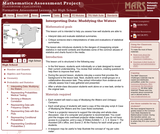Rating

This lesson unit is intended to help teachers assess how well students are able to: interpret data and evaluate statistical summaries; and critique someone elseŐs interpretations of data and evaluations of statistical summaries. The lesson also introduces students to the dangers of misapplying simple statistics in real-world contexts, and illustrates some of the common abuses of statistics and charts found in the media.

Subject:
Statistics and Probability
Material Type:
Assessment
Lesson Plan
Provider:
Shell Center for Mathematical Education
U.C. Berkeley
Provider Set:
Mathematics Assessment Project (MAP)
Mathematics Assessment Project (MAP)
Date Added:
04/26/2013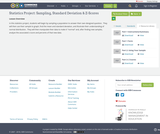Conditions of Use:
Remix and Share
Rating

In this statistics project, students will begin by sampling a population to answer their own designed question.  They will then use their sample to graph, find the mean and standard deviation, and illustrate their understanding of normal distribution.  They will then manipulate their data to make it "normal" and, after finding new samples, analyze the associated z-score and percents of that new data.

Subject:
Statistics and Probability
Material Type:
Lesson Plan
Author:
Melissa Hesterman
Date Added:
12/07/2017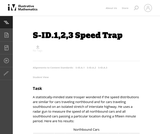Conditions of Use:
Remix and Share
Rating

The purpose of this task is to allow students to demonstrate an ability to construct boxplots and to use boxplots as the basis for comparing distributions. The solution should directly compare the center, spread, and shape of the two distributions and comment on the high outlier in the northbound data set.

Subject:
Mathematics
Material Type:
Activity/Lab
Provider:
Illustrative Mathematics
Provider Set:
Illustrative Mathematics
Author:
Illustrative Mathematics
Date Added:
02/12/2013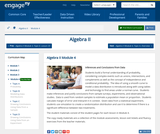Conditions of Use:
Remix and Share
Rating

Students build a formal understanding of probability, considering complex events such as unions, intersections, and complements as well as the concept of independence and conditional probability.  The idea of using a smooth curve to model a data distribution is introduced along with using tables and techonolgy to find areas under a normal curve.  Students make inferences and justify conclusions from sample surveys, experiments, and observational studies.  Data is used from random samples to estimate a population mean or proportion.  Students calculate margin of error and interpret it in context.  Given data from a statistical experiment, students use simulation to create a randomization distribution and use it to determine if there is a significant difference between two treatments.

Subject:
Algebra
Material Type:
Module
Provider:
New York State Education Department
Provider Set:
EngageNY
Date Added:
03/24/2016